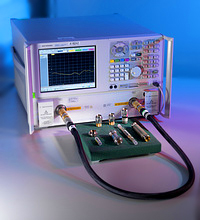Close
Close

# Impedance standards

## Measuring impedance is key to all measurements at RF and microwave frequencies

The measurement of impedance may be expressed in a number of ways depending upon the requirement. In a standards environment, it is most useful to give the scattering parameters or the voltage reflection or transmission coefficient that would apply if an item were connected to the ideal of the medium of interest.The impedance of a simple transmission line can, in principle, be calculated in terms of its dimensions and the materials of which it has been constructed. However, the uncertainties involved in such a calculation increase rapidly with the number of different materials involved in the construction and the complexity of the electromagnetic fields.

For simple structures, such as coaxial lines or rectangular waveguides, impedance standards can be constructed whose behaviour can be calculated in terms of the fundamental SI quantities of mass, length, time and current. Examples of these standards are air lines, waveguide spacers and plain short-circuits. There is also a second class of standards whose behaviour is predictable but not strictly calculable, e.g. open-circuits with collet depressors and offset short-circuits.

Impedance measurements are performed using a vector network analyser (VNA).  In order to perform such a measurement, the VNA must first be calibrated. There are a number of methods one can use to calibrate a VNA and it is this calibration that ultimately determines the uncertainty of the measurement.

By using the Through-Reflect-Line (TRL) technique to calibrate the VNA, NPL can provide the lowest possible uncertainty of measurement. The use of air lines in the TRL method ensures traceability to SI quantities through the dimensional measurements of the air line standards used during the calibration process.

The TRL scheme has its limits and starts to become unusable at lower frequencies as the length of air line required becomes impractical. At these frequencies, NPL uses a Short-Open-Load (SOL) technique to calibrate the VNA. The standards are characterized via their electrical properties at DC and RF frequencies and these characteristics and uncertainties are propagated through the calibration to ensure accuracy and traceability.

Most users will want to verify their VNA calibration and this is done using a verification kit, which will contain a number of devices that have been (traceably) measured so the user knows what to expect when the devices are connected to the VNA. NPL is a key provider of such measurements for accredited laboratories throughout the world.

All of NPL’s impedance measurements are valid under the CIPM’s Mutual Recognition Agreement, which requires that measurements are traceable to the SI, and thus satisfies the requirements of ISO 17025.